# RS Aggarwal Solutions for Class 9 Chapter 17: Bar Graph, Histogram and Frequency Polygon Exercise 17A

## RS Aggarwal Solutions for Class 9 Maths Exercise 17A PDF

This is the first exercise of Chapter 17 which contains problems solved on representing the given data using bar graphs. This is one of the scoring concepts in Mathematics as the students can obtain a good score in the board exam based on its weightage. The students by understanding the concept and formulas can solve huge numbers of problems in a shorter duration. The solutions are created by subject experts with the aim of making the concepts easier for students. RS Aggarwal Solutions for Class 9 Maths Chapter 17 Bar Graph, Histogram and Frequency Polygon Exercise 17A are provided here.

## RS Aggarwal Solutions for Class 9 Chapter 17: Bar Graph, Histogram and Frequency Polygon Exercise 17A Download PDF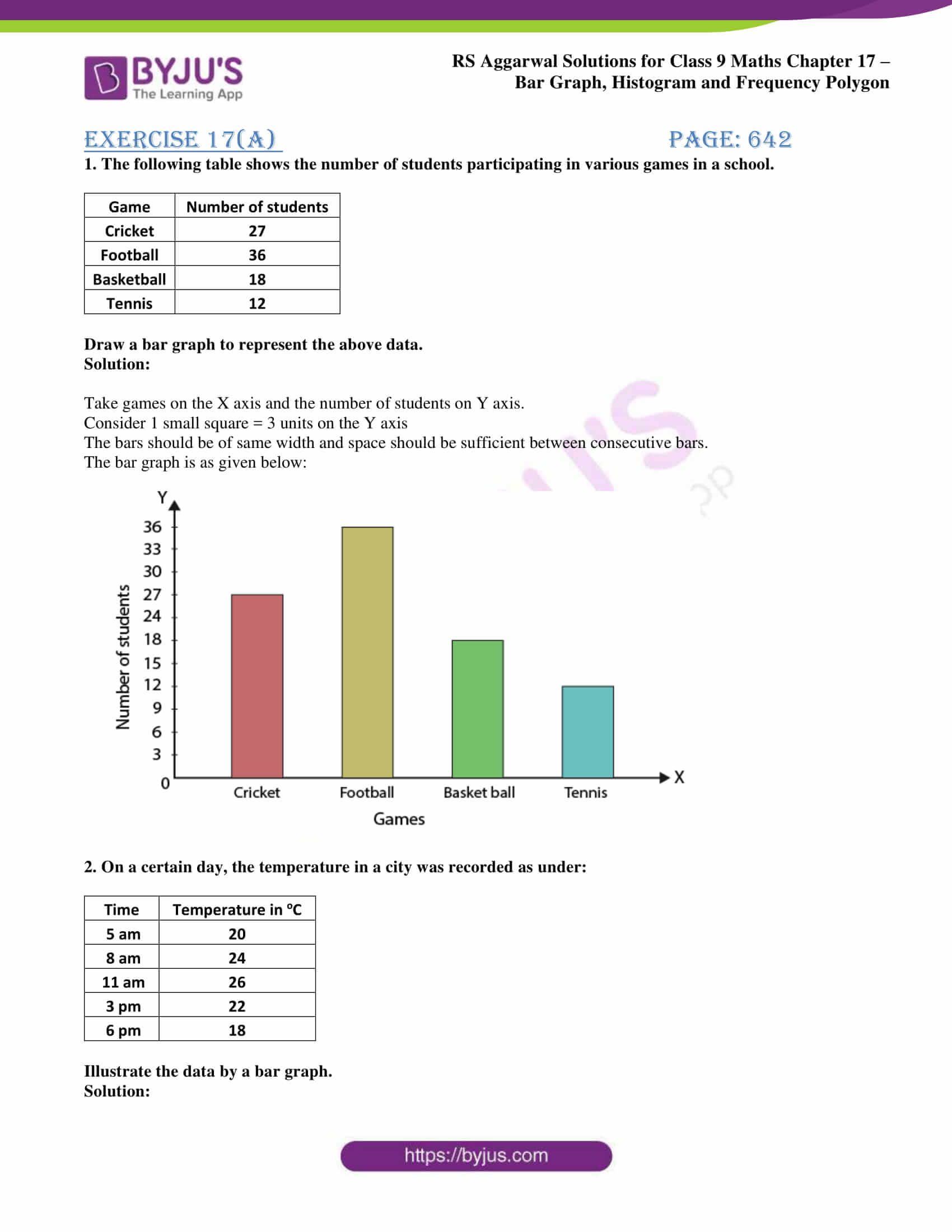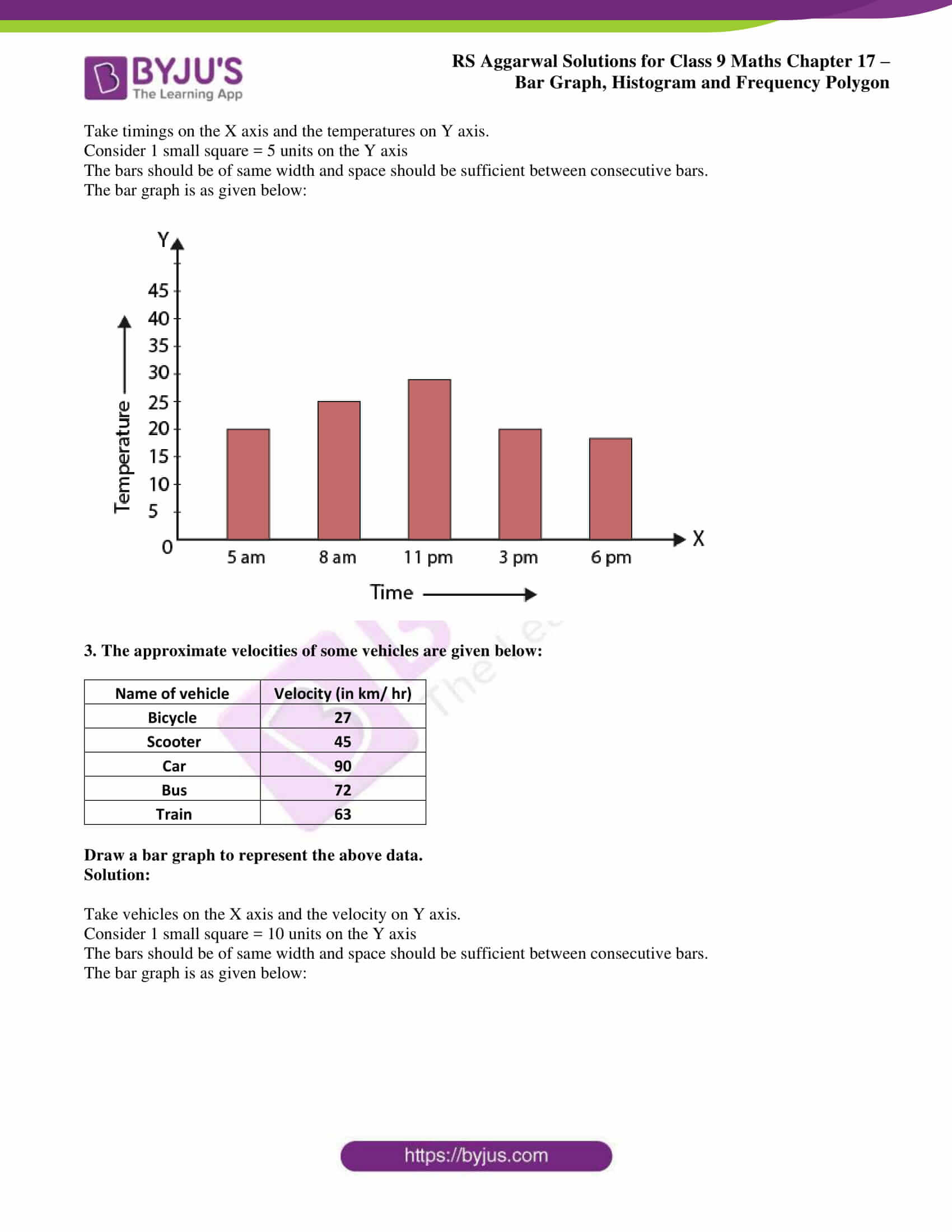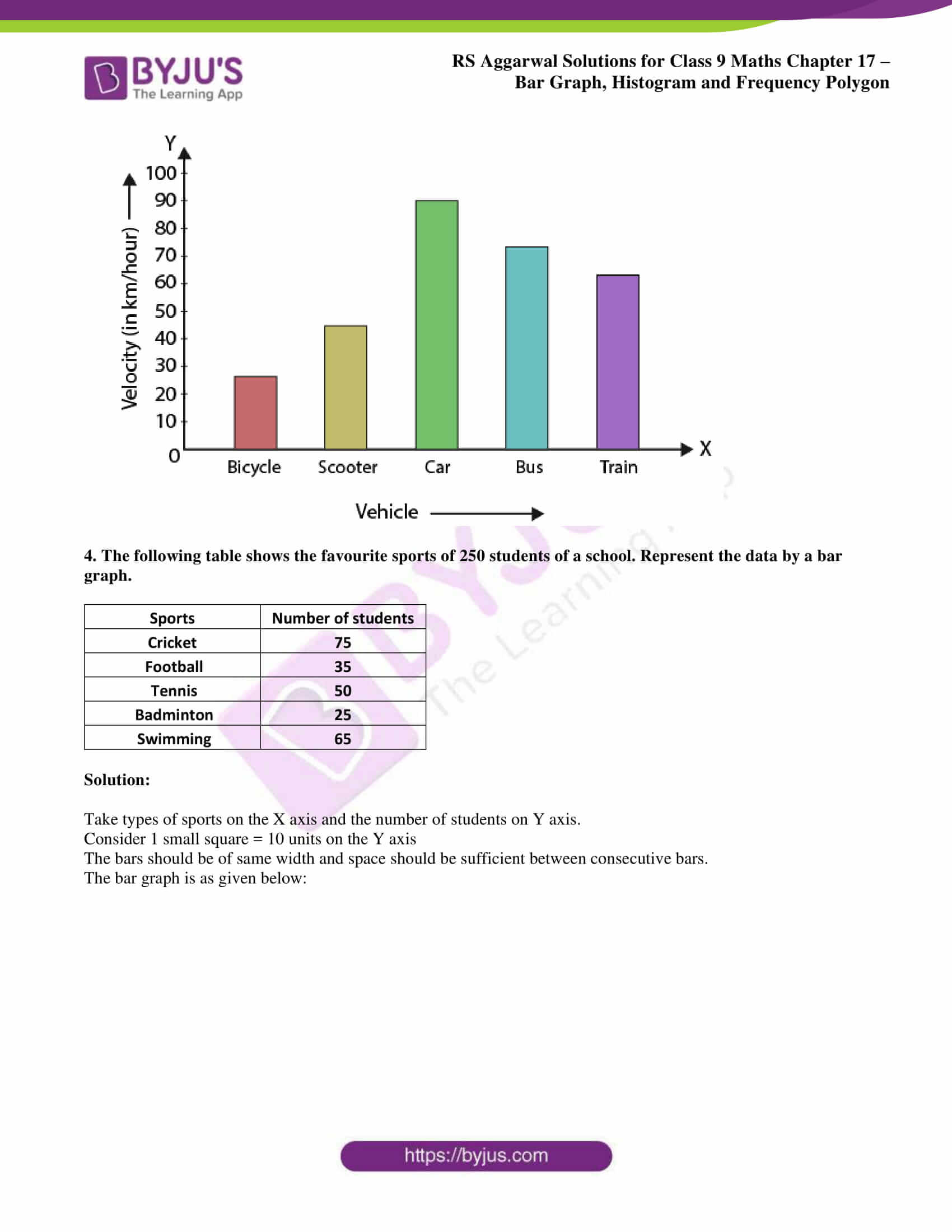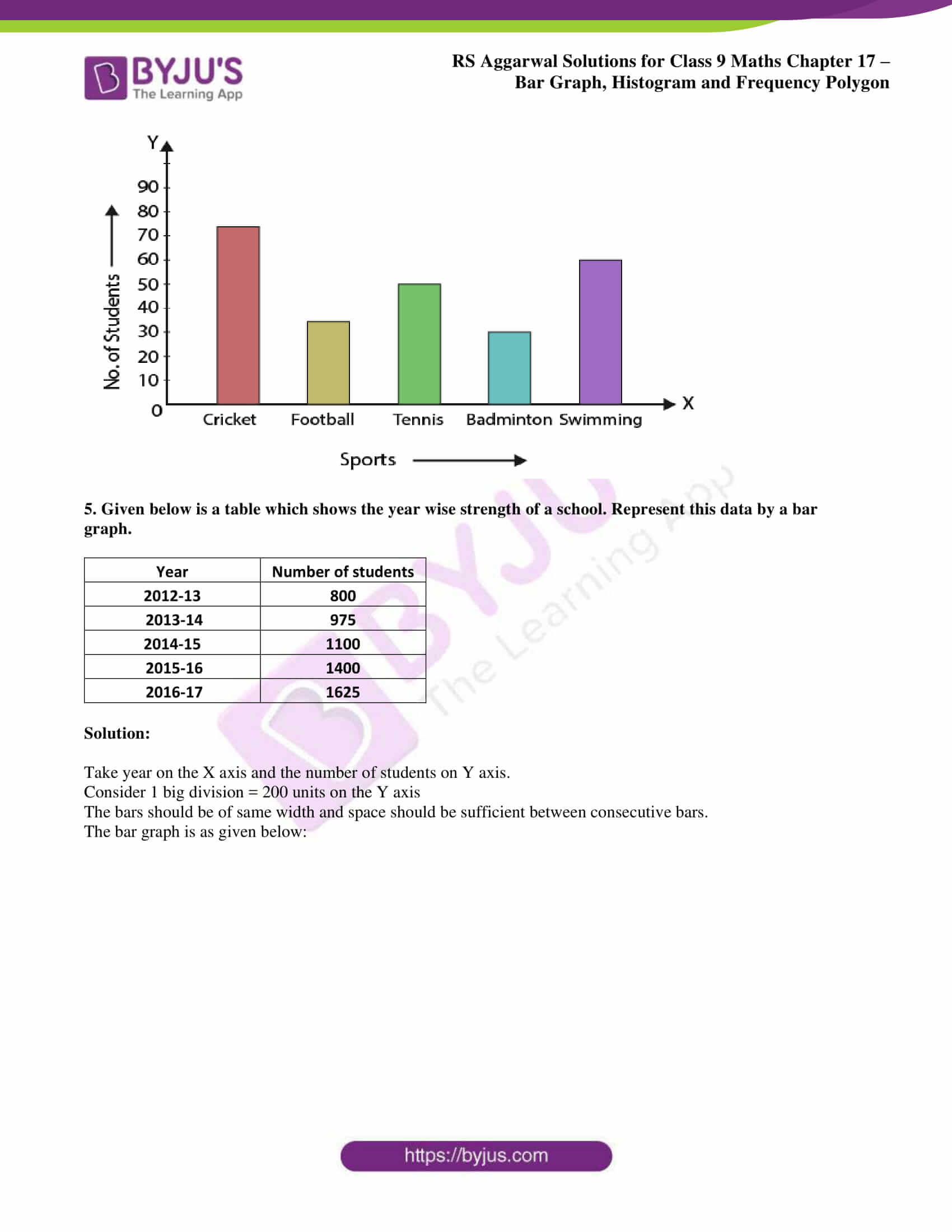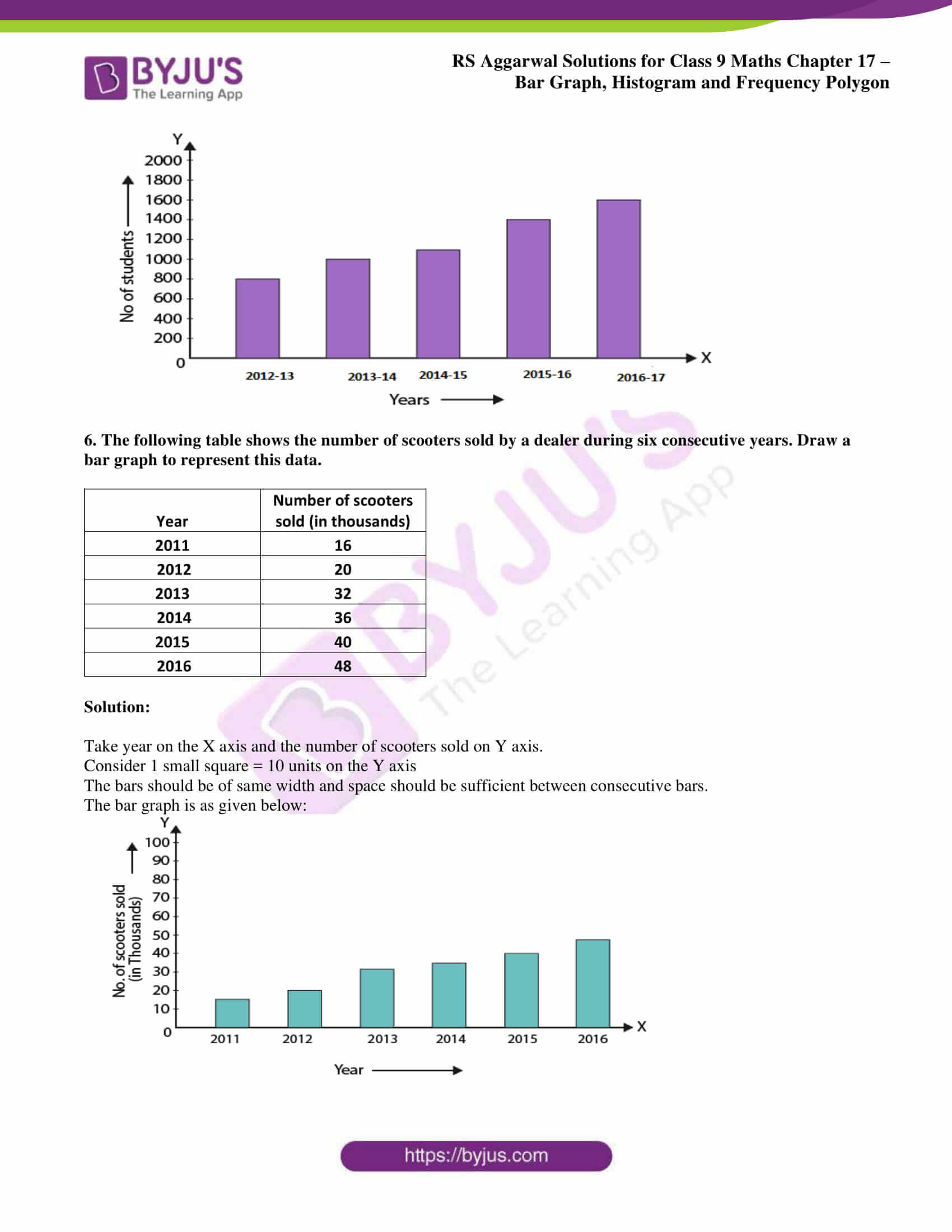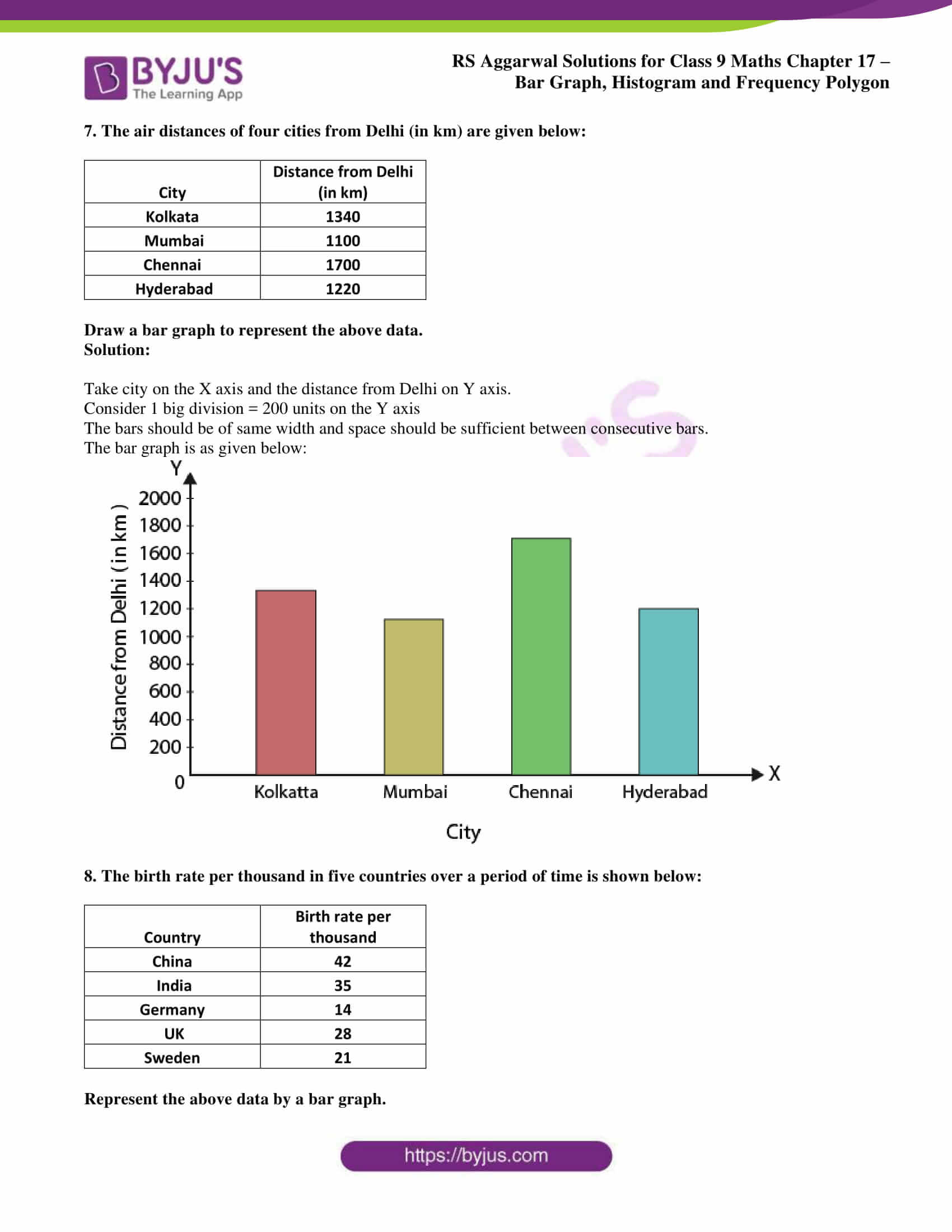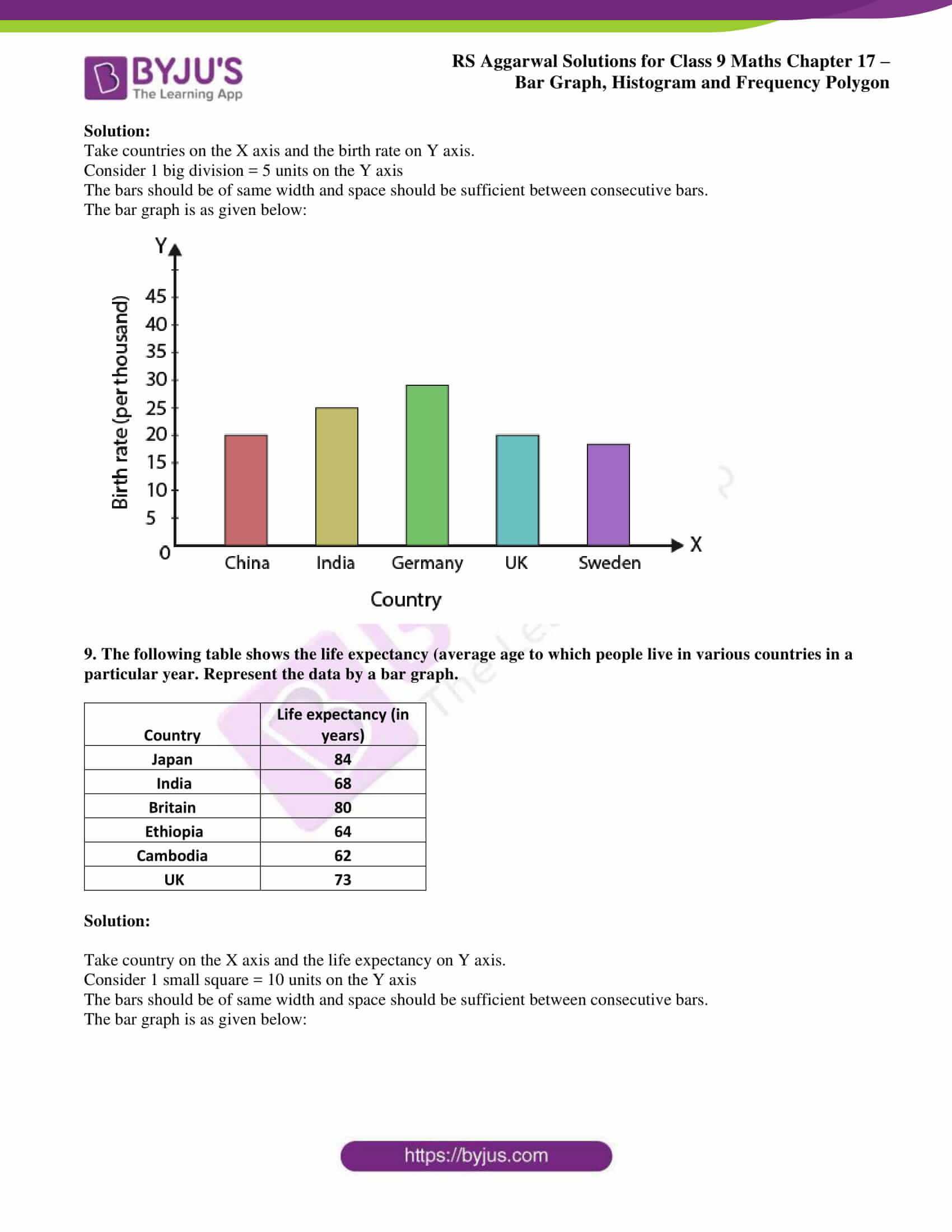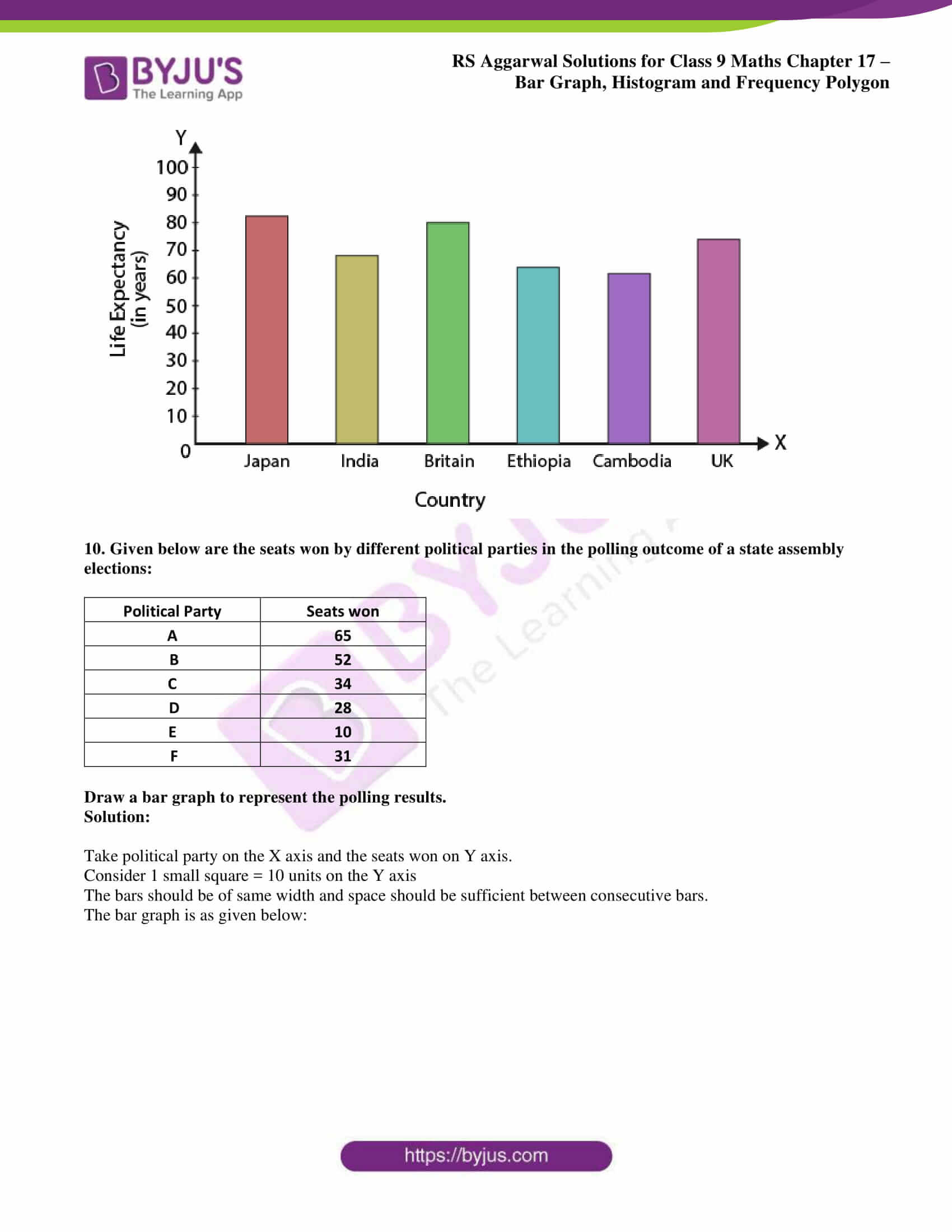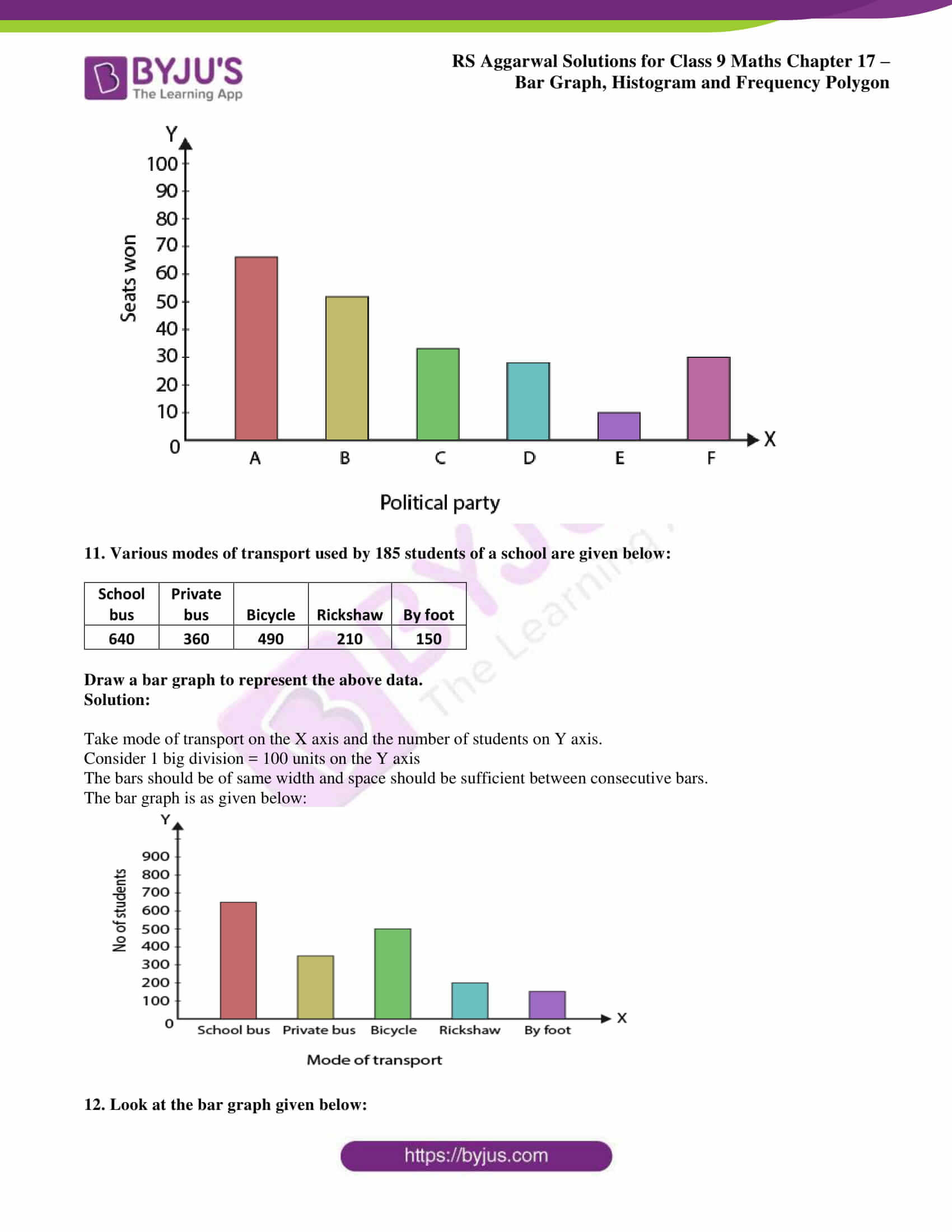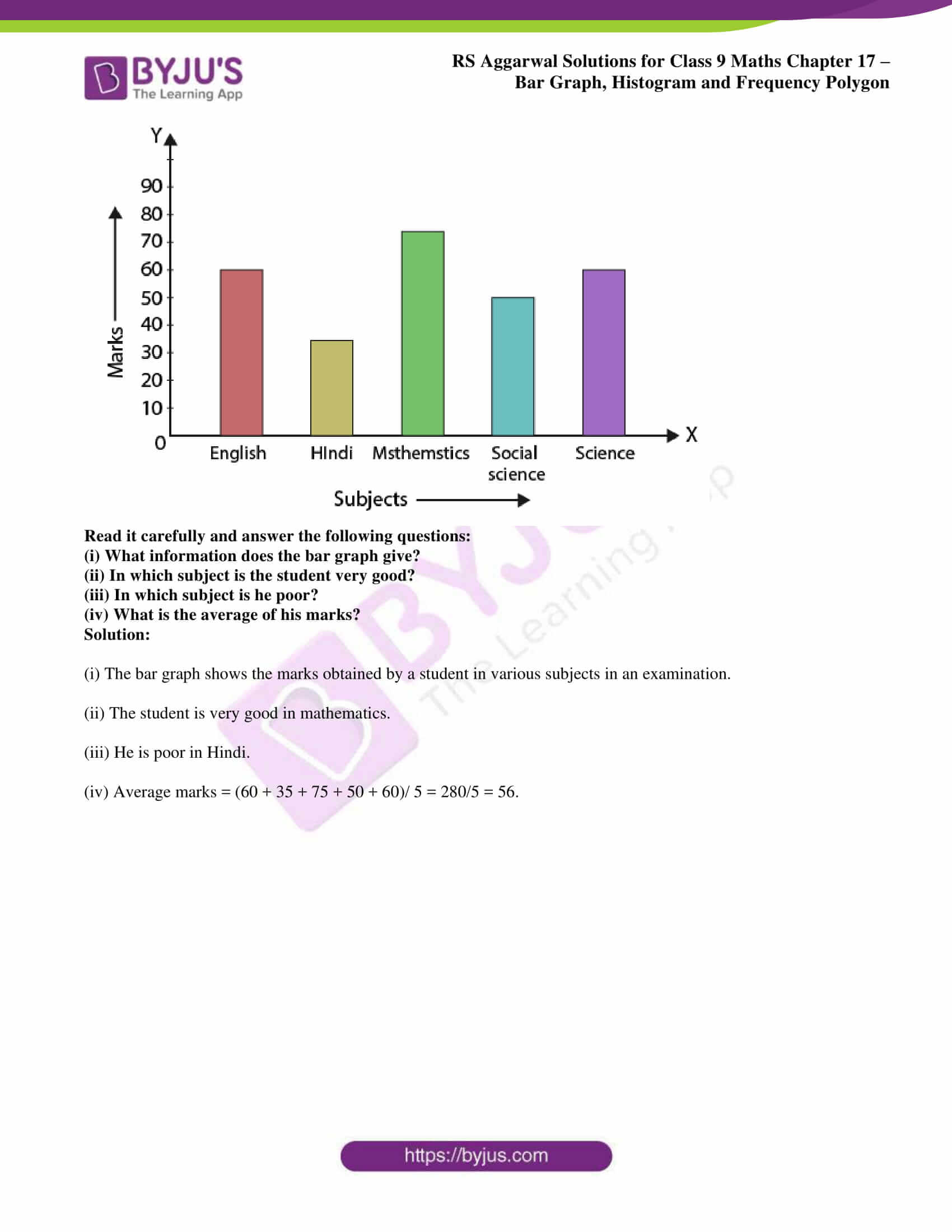## Access RS Aggarwal Solutions for Class 9 Chapter 17: Bar Graph, Histogram and Frequency Polygon Exercise 17A

### Exercise 17(A) page: 642

1. The following table shows the number of students participating in various games in a school.

 Game Number of students Cricket 27 Football 36 Basketball 18 Tennis 12

Draw a bar graph to represent the above data.

Solution:

Take games on the X axis and the number of students on Y axis.

Consider 1 small square = 3 units on the Y axis

The bars should be of same width and space should be sufficient between consecutive bars.

The bar graph is as given below: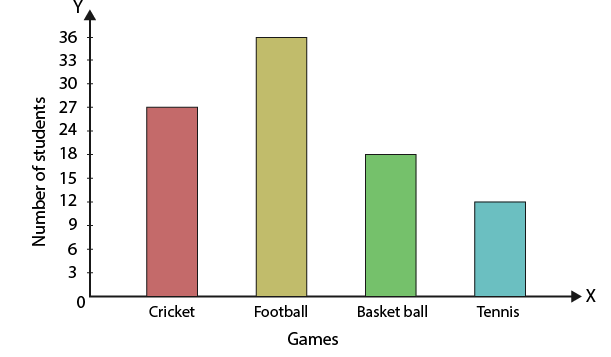2. On a certain day, the temperature in a city was recorded as under:

 Time Temperature in oC 5 am 20 8 am 24 11 am 26 3 pm 22 6 pm 18

Illustrate the data by a bar graph.

Solution:

Take timings on the X axis and the temperatures on Y axis.

Consider 1 small square = 5 units on the Y axis

The bars should be of same width and space should be sufficient between consecutive bars.

The bar graph is as given below: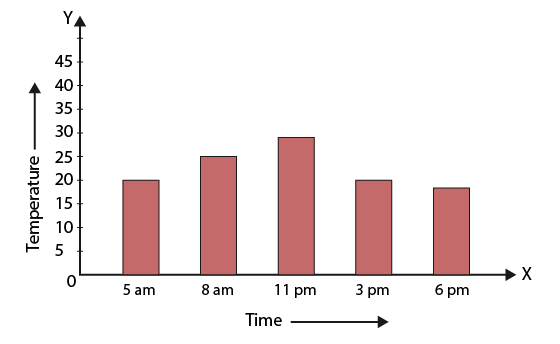3. The approximate velocities of some vehicles are given below:

 Name of vehicle Velocity (in km/ hr) Bicycle 27 Scooter 45 Car 90 Bus 72 Train 63

Draw a bar graph to represent the above data.

Solution:

Take vehicles on the X axis and the velocity on Y axis.

Consider 1 small square = 10 units on the Y axis

The bars should be of same width and space should be sufficient between consecutive bars.

The bar graph is as given below: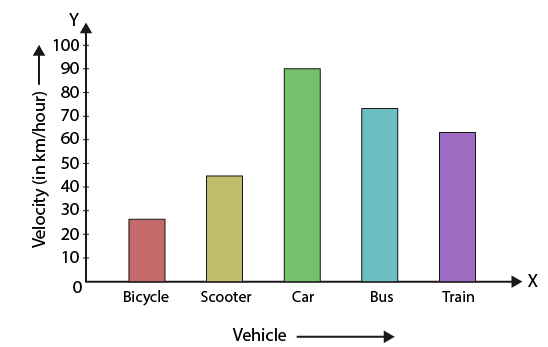4. The following table shows the favourite sports of 250 students of a school. Represent the data by a bar graph.

 Sports Number of students Cricket 75 Football 35 Tennis 50 Badminton 25 Swimming 65

Solution:

Take types of sports on the X axis and the number of students on Y axis.

Consider 1 small square = 10 units on the Y axis

The bars should be of same width and space should be sufficient between consecutive bars.

The bar graph is as given below: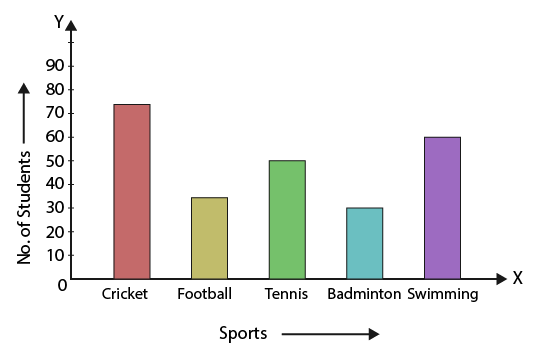5. Given below is a table which shows the year wise strength of a school. Represent this data by a bar graph.

 Year Number of students 2012-13 800 2013-14 975 2014-15 1100 2015-16 1400 2016-17 1625

Solution:

Take year on the X axis and the number of students on Y axis.

Consider 1 big division = 200 units on the Y axis

The bars should be of same width and space should be sufficient between consecutive bars.

The bar graph is as given below: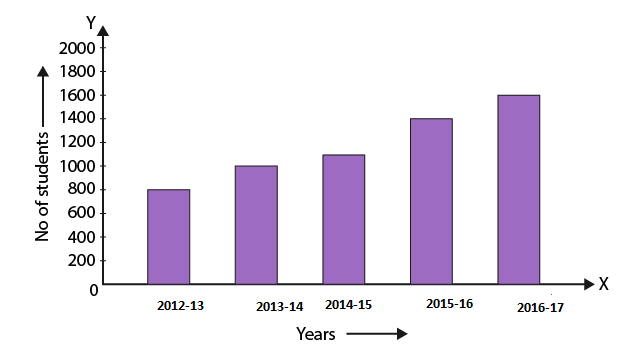6. The following table shows the number of scooters sold by a dealer during six consecutive years. Draw a bar graph to represent this data.

 Year Number of scooters sold (in thousands) 2011 16 2012 20 2013 32 2014 36 2015 40 2016 48

Solution:

Take year on the X axis and the number of scooters sold on Y axis.

Consider 1 small square = 10 units on the Y axis

The bars should be of same width and space should be sufficient between consecutive bars.

The bar graph is as given below: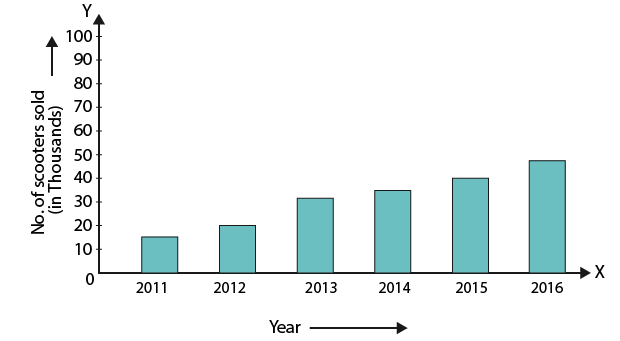7. The air distances of four cities from Delhi (in km) are given below:

 City Distance from Delhi (in km) Kolkata 1340 Mumbai 1100 Chennai 1700 Hyderabad 1220

Draw a bar graph to represent the above data.

Solution:

Take city on the X axis and the distance from Delhi on Y axis.

Consider 1 big division = 200 units on the Y axis

The bars should be of same width and space should be sufficient between consecutive bars.

The bar graph is as given below: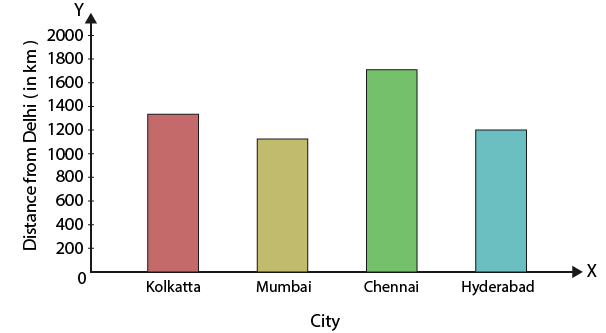8. The birth rate per thousand in five countries over a period of time is shown below:

 Country Birth rate per thousand China 42 India 35 Germany 14 UK 28 Sweden 21

Represent the above data by a bar graph.

Solution:

Take countries on the X axis and the birth rate on Y axis.

Consider 1 big division = 5 units on the Y axis

The bars should be of same width and space should be sufficient between consecutive bars.

The bar graph is as given below: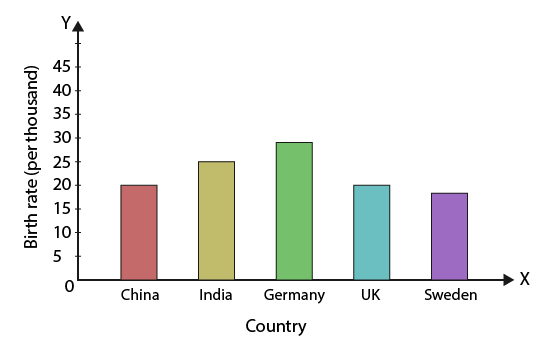9. The following table shows the life expectancy (average age to which people live in various countries in a particular year. Represent the data by a bar graph.

 Country Life expectancy (in years) Japan 84 India 68 Britain 80 Ethiopia 64 Cambodia 62 UK 73

Solution:

Take country on the X axis and the life expectancy on Y axis.

Consider 1 small square = 10 units on the Y axis

The bars should be of same width and space should be sufficient between consecutive bars.

The bar graph is as given below: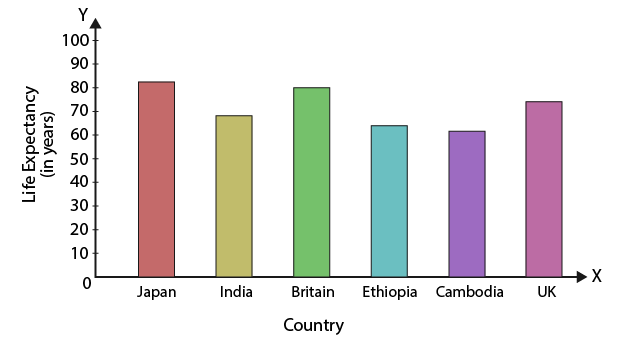10. Given below are the seats won by different political parties in the polling outcome of a state assembly elections:

 Political Party Seats won A 65 B 52 C 34 D 28 E 10 F 31

Draw a bar graph to represent the polling results.

Solution:

Take political party on the X axis and the seats won on Y axis.

Consider 1 small square = 10 units on the Y axis

The bars should be of same width and space should be sufficient between consecutive bars.

The bar graph is as given below: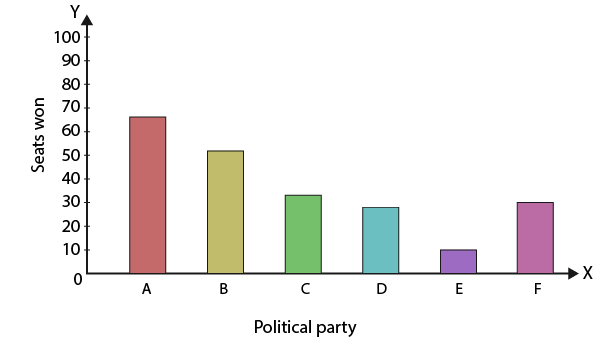11. Various modes of transport used by 185 students of a school are given below:

 School bus Private bus Bicycle Rickshaw By foot 640 360 490 210 150

Draw a bar graph to represent the above data.

Solution:

Take mode of transport on the X axis and the number of students on Y axis.

Consider 1 big division = 100 units on the Y axis

The bars should be of same width and space should be sufficient between consecutive bars.

The bar graph is as given below: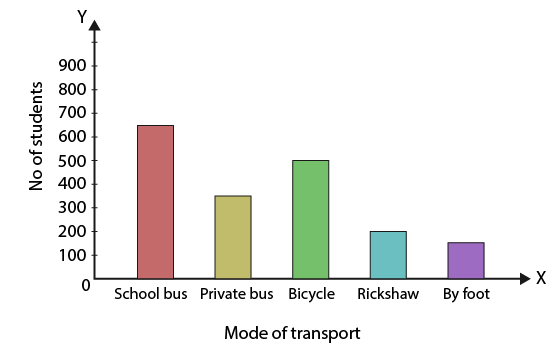12. Look at the bar graph given below: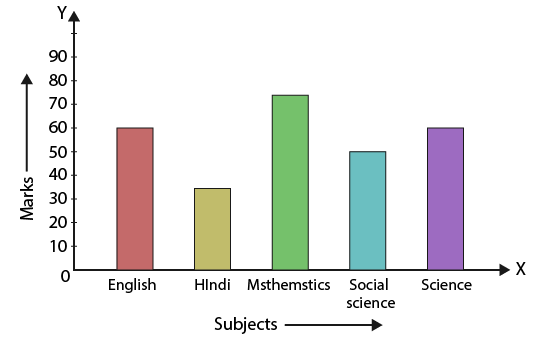(i) What information does the bar graph give?

(ii) In which subject is the student very good?

(iii) In which subject is he poor?

(iv) What is the average of his marks?

Solution:

(i) The bar graph shows the marks obtained by a student in various subjects in an examination.

(ii) The student is very good in mathematics.

(iii) He is poor in Hindi.

(iv) Average marks = (60 + 35 + 75 + 50 + 60)/ 5 = 280/5 = 56.

### Access other exercise solutions of Class 9 Maths Chapter 17: Bar Graph, Histogram and Frequency Polygon

Exercise 17B Solutions 16 Questions

### RS Aggarwal Solutions Class 9 Maths Chapter 17 – Bar Graph, Histogram and Frequency Polygon Exercise 17A

RS Aggarwal Solutions Class 9 Maths Chapter 17 Bar Graph, Histogram and Frequency Polygon Exercise 17A explains the graphical representation of given data with the help of a bar graph.

### Key features of RS Aggarwal Solutions for Class 9 Maths Chapter 17: Bar Graph, Histogram and Frequency Polygon Exercise 17A

• RS Aggarwal Solutions help students to realize their areas of weaknesses and identify the time required to improve upon them.
• By solving examples and exercise wise problems using the solutions created by our experts, students can obtain a good academic score.
• Tips and shortcuts are explained which help students to solve tricky problems faster in the exam.
• Conceptual understanding is enhanced among students, which is the main aim of preparing solutions.